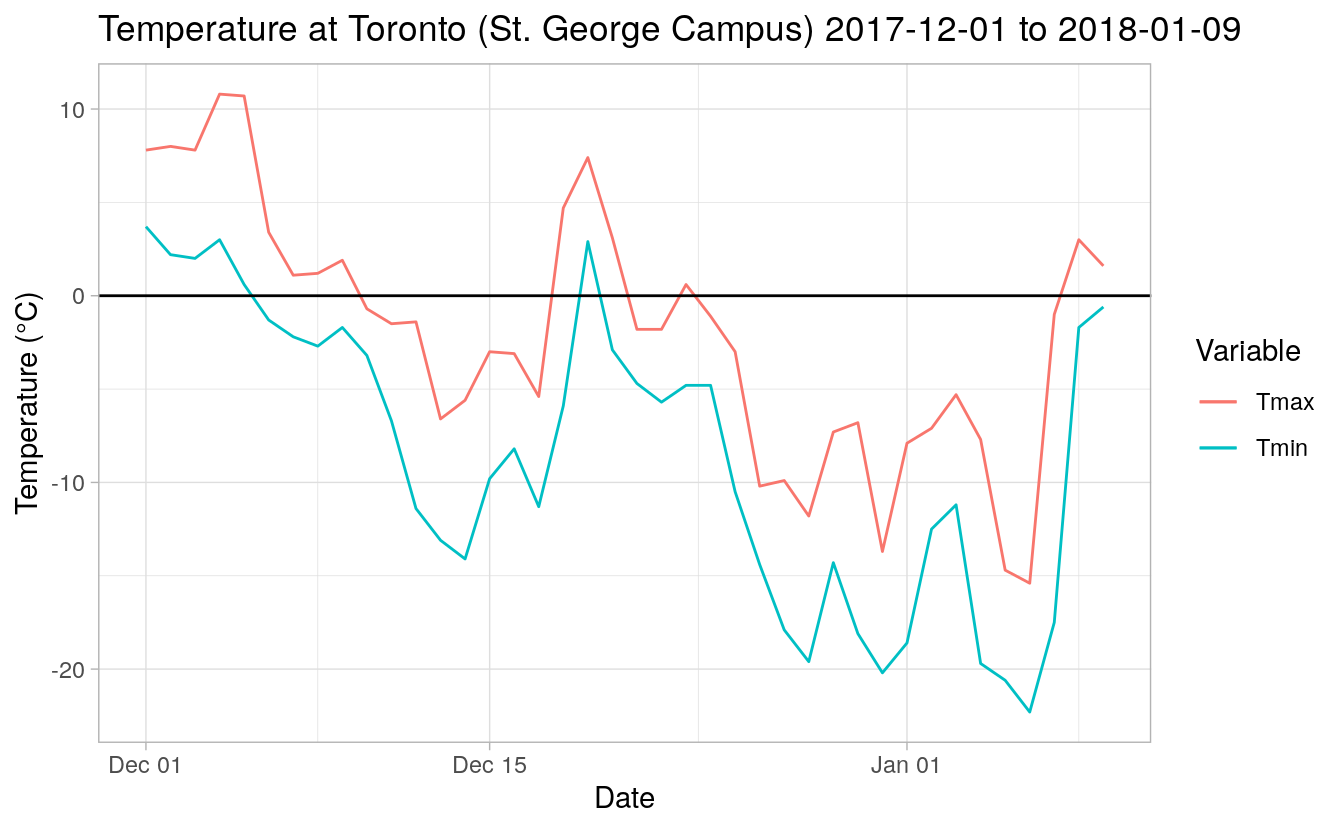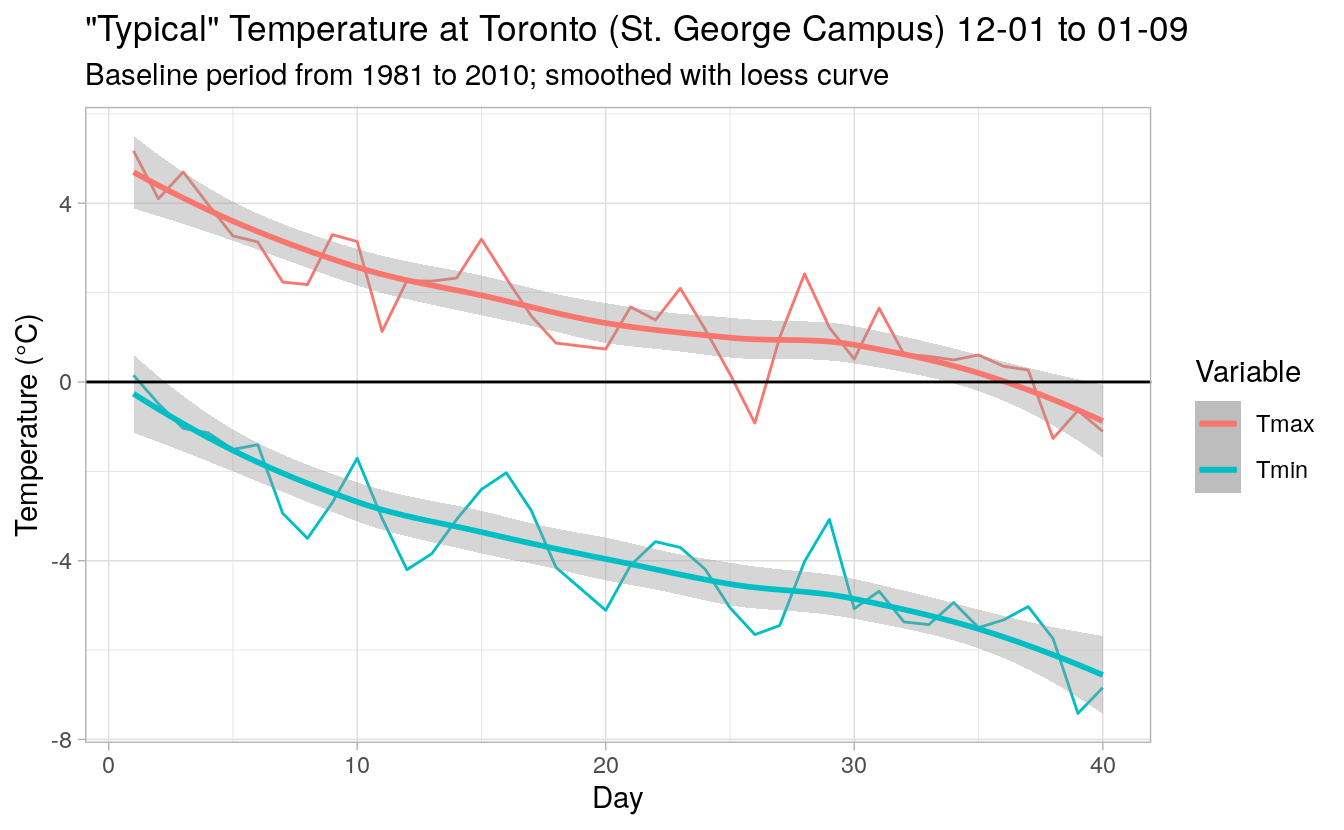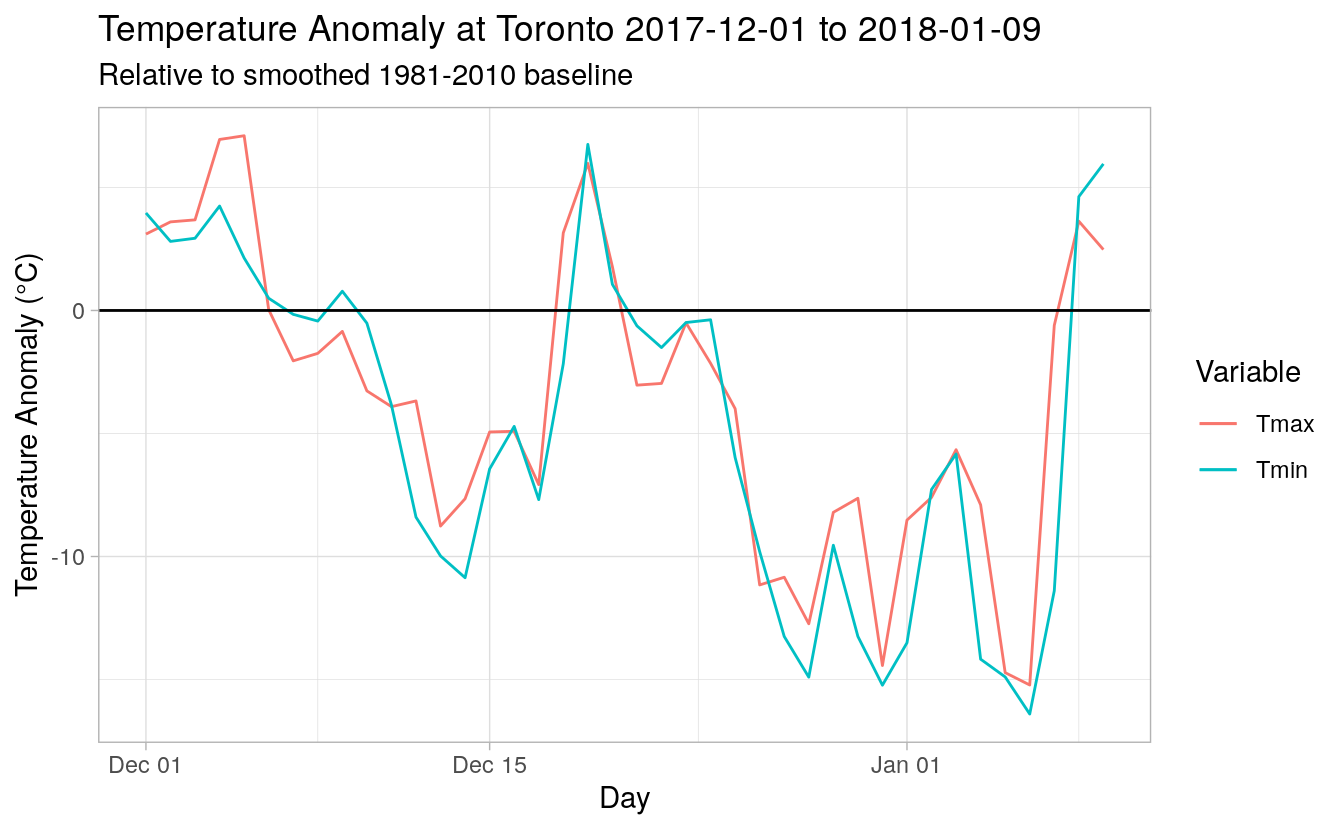# Revisiting December Temperatures at Toronto

## Introduction

In Anderson and Gough ( 2017), Bill Gough and I examined winter temperatures in Toronto, with a special focus on winters 2013/14 and 2014/15. With the recent cold snap, I thought it would be interesting to re-examine the subject. This will all be done in R, with the full code included in this blog post.

First, I will load the libraries that I will use.

library(canadaHCDx)
library(dplyr)
library(ggplot2)
library(tibble)
library(tidyr)
library(zoo)


Now I get the Toronto temperature data:

# Toronto changed station code afer June 2003, so we'll merge it with the new one.
tor <- hcd_daily(5051, 1840:2003)
tmp <- hcd_daily(31688, 2003:2018)
tor <- bind_rows(tor[1:which(tor$Date == "2003-06-30"),], tmp[which(tmp$Date == "2003-07-01"):which(tmp$Date == "2018-01-31"),])  ## Plots Let’s first see what the temperature looked like. First, I prepare some “plot data”, extracting December 01 to January 09 (last day of available data so far in 2018). plot_data <- tor %>% select(Date, MaxTemp, MinTemp, MeanTemp) %>% filter(Date >= "2017-12-01" & Date <= "2018-01-09")  Let’s see what that looks like: ggplot(data = plot_data) + geom_line(aes(Date, MaxTemp, col = "Tmax")) + geom_line(aes(Date, MinTemp, col = "Tmin")) + geom_hline(aes(yintercept = 0)) + labs(x = "Date", y = "Temperature (°C)", color = "Variable") + ggtitle("Temperature at Toronto (St. George Campus) 2017-12-01 to 2018-01-09") + theme_light()We can see that the beginning of December was fairly mild, with an additional mild period around the 18th to 20th before plummeting in the last days of 2017 and beginning of 2018. ## Comparison to daily “normals” We can’t say for sure that weather is anomalous, unless we compare it to normalized data. Let’s see how December and January look, by plotting the “normal” temperature, i.e. the 30-year average for the same period for each day from 1981–2010. First, I will generate daily normals by averaging each calendar date over the past 30 years. Note that I am not sure whether this is sloppy methodology, but thought I’d give it a shot. tor_norm <- tor %>% select(Date, MaxTemp, MinTemp, MeanTemp) %>% filter(format(Date, format = "%Y") >= 1981 & format(Date, format = "%Y") <= 2010) tor_norm <- tor_norm %>% group_by(Day = format(Date, format = "%m-%d")) %>% summarize_all(mean, na.rm = TRUE) %>% select(-Date)  Now that I have the 30-year daily average temperature for each day of the year, I’ll extract just December 1 to January 9. I’ll plot these with a loess curve and use the values from that curve to represent my “typical” temperature. tor_typical <- bind_rows(tor_norm[tor_norm$Day >= "12-01",], tor_norm[tor_norm$Day <= "01-09",]) %>% add_column(Index = 1:nrow(.)) %>% add_column(MaxSmooth = predict(loess(MaxTemp~Index, .), .$Index), MinSmooth = predict(loess(MinTemp~Index, .), .$Index)) ggplot(data = tor_typical) + geom_line(aes(1:40, MaxTemp, col = "Tmax")) + geom_smooth(aes(1:40, MaxTemp, col = "Tmax")) + geom_line(aes(1:40, MinTemp, col = "Tmin")) + geom_smooth(aes(1:40, MinTemp, col = "Tmin")) + geom_hline(aes(yintercept = 0)) + labs(x = "Day", y = "Temperature (°C)", color = "Variable") + ggtitle("\"Typical\" Temperature at Toronto (St. George Campus) 12-01 to 01-09", subtitle = "Baseline period from 1981 to 2010; smoothed with loess curve") + theme_light()Now let’s compare our temperature with the “typical”. plot_data <- plot_data %>% add_column(MaxTempAnom = (.$MaxTemp - tor_typical$MaxSmooth), MinTempAnom = (.$MinTemp - tor_typical$MinSmooth)) ggplot(data = plot_data) + geom_line(aes(Date, MaxTempAnom, col = "Tmax")) + geom_line(aes(Date, MinTempAnom, col = "Tmin")) + geom_hline(aes(yintercept = 0))+ labs(x = "Day", y = "Temperature Anomaly (°C)", color = "Variable") + ggtitle("Temperature Anomaly at Toronto 2017-12-01 to 2018-01-09", subtitle = "Relative to smoothed 1981-2010 baseline") + theme_light()Indeed, temperatures were cold, getting to be as much as 16 °C colder than the smoothed average daily temperature from 1981–2010. ## How does December rank? Now let’s prepare some December-only data series at the daily and monthly timescales. tor_dec <- tor %>% select(Date, MaxTemp, MinTemp, MeanTemp) %>% filter(format(Date, format = "%m") == 12) tor_dec_mon <- tor_dec %>% group_by(Yearmon = format(Date, format = "%Y-%m")) %>% summarize_all(mean, na.rm = TRUE)  Note, I have become largely familiar with the Toronto data series, and I know that missing data is not an issue in this time series. Generally, one would need to do a quick check of the data, such as: quick_audit(tor_dec, c("MaxTemp", "MinTemp", "MeanTemp"), by = "month") #> # A tibble: 178 x 6 #> Year Month Year-month MaxTemp pct NA MinTemp pct NA MeanTemp pct NA #> <chr> <chr> <yearmon> <dbl> <dbl> <dbl> #> 1 1840 12 Dec 1840 0 0 0 #> 2 1841 12 Dec 1841 0 0 0 #> 3 1842 12 Dec 1842 0 0 0 #> 4 1843 12 Dec 1843 0 0 0 #> 5 1844 12 Dec 1844 0 0 0 #> 6 1845 12 Dec 1845 0 0 0 #> 7 1846 12 Dec 1846 0 0 0 #> 8 1847 12 Dec 1847 0 0 0 #> 9 1848 12 Dec 1848 0 0 0 #> 10 1849 12 Dec 1849 0 0 0 #> # … with 168 more rows  OK, let’s look at some monthly ranks! tor_dec_mon <- tor_dec_mon %>% add_column(MaxTempRank = min_rank(.$MaxTemp), MinTempRank = min_rank(.$MinTemp), MeanTempRank = min_rank(.$MeanTemp))

tail(tor_dec_mon)

#> # A tibble: 6 x 8
#>   Yearmon Date       MaxTemp MinTemp MeanTemp MaxTempRank MinTempRank
#>   <chr>   <date>       <dbl>   <dbl>    <dbl>       <int>       <int>
#> 1 2012-12 2012-12-16   4.76   -0.827    1.94          171         173
#> 2 2013-12 2013-12-16  -0.567  -5.60    -3.24           45          81
#> 3 2014-12 2014-12-16   3.25   -1.57     0.857         148         163
#> 4 2015-12 2015-12-16   7.57    2.52     5.06          178         178
#> 5 2016-12 2016-12-16   2.23   -2.97    -0.387         125         139
#> 6 2017-12 2017-12-16  -0.845  -6.94    -3.91           36          46
#> # … with 1 more variable: MeanTempRank <int>


Toronto’s temperature in the month of December 2017 was ranked 36 for $T_{max}$ (-0.845 °C with a return interval (RI) of 4.97) , 46 for $T_{min}$ (-6.94 °C with RI of 3.89), and 40 for $T_{mean}$ (-3.91 °C with RI of 4.47). All three variables are ranked out of 178 total Decembers in the set.

The last time that we saw a December this cold was in 2000-12. The temperature that year was:

• $T_{max}$: -2.11 °C, rank 12, with RI of 14.9
• $T_{min}$: -7.73 °C, rank 34, with RI of 5.26
• $T_{mean}$: -4.94 °C, rank 24, with RI of 7.46

The second-last December that was as cold or colder than December 2017 was 1989-12. That year the temperature reached:

• $T_{max}$: -4.63 °C, rank 1, with RI of 179
• $T_{min}$: -10.9 °C, rank 3, with RI of 59.7
• $T_{mean}$: -7.79 °C, rank 3, with RI of 59.7

## Air Mass Analysis

One of the things that we looked at in our 2017 paper was the position of the jet stream. During December 2017, we know that the jet stream was to the south of Toronto during the cold snap. Let’s look at this, using data from Sheridan’s Spatial Synoptic Classification (SSC) (Sheridan 2002).

First, I’ll get the data and bin it into four bins, as per Anderson and Gough ( 2017):

1. ‘cool’: SSC codes 2 (dry polar) and 5 (moist polar)
2. ‘moderate’: SSC codes 1 (dry moderate) and 4 (moist moderate)
3. ‘warm’: SSC codes 3 (dry tropical), 6 (moist tropical), 66 (moist tropical plus), and 67 (moist tropical double plus)
4. ‘transition’: SCC code 7 (transition)
f <- tempfile()